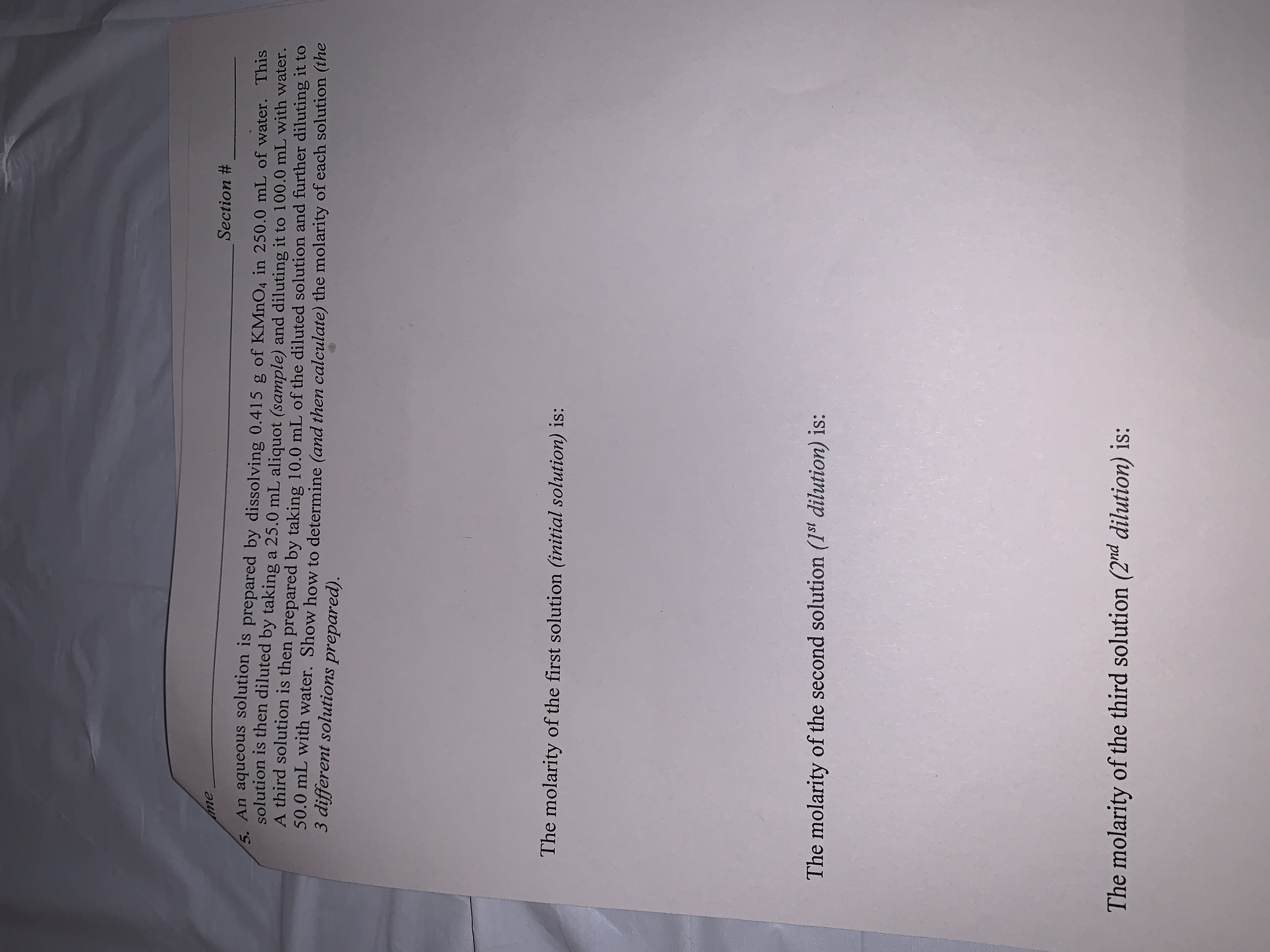# meSection #5. An aqueous solution is prepared by dissolving 0.415 g of KMnO4 in 250.0 mL of water. Thissolution is then diluted by taking a 25.0 mL aliquot (sample) and diluting it to 100.0 mL with water.A third solution is then prepared by taking 10.0 mL of the diluted solution and further diluting it to50.0 mL with water. Show how to determine (and then calculate) the molarity of each solution (the3 different solutions prepared).The molarity of the first solution (initial solution) is:The molarity of the second solution (1st dilution) is:The molarity of the third solution (2nd dilution) is:

Question
6 views

Chem 1121help_outlineImage Transcriptioncloseme Section # 5. An aqueous solution is prepared by dissolving 0.415 g of KMnO4 in 250.0 mL of water. This solution is then diluted by taking a 25.0 mL aliquot (sample) and diluting it to 100.0 mL with water. A third solution is then prepared by taking 10.0 mL of the diluted solution and further diluting it to 50.0 mL with water. Show how to determine (and then calculate) the molarity of each solution (the 3 different solutions prepared). The molarity of the first solution (initial solution) is: The molarity of the second solution (1st dilution) is: The molarity of the third solution (2nd dilution) is: fullscreen
check_circle

Step 1

Molarity is given by,

Molarity = mole/liter

The concentration of diluted solution is calculated by using volumetric formula, it is

Step 2

Molarity of first solution is calculated as,

Step 3

Molarity of second solutio...

### Want to see the full answer?

See Solution

#### Want to see this answer and more?

Solutions are written by subject experts who are available 24/7. Questions are typically answered within 1 hour.*

See Solution
*Response times may vary by subject and question.
Tagged in

### Chemistry